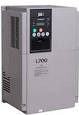# 440 VOLT THREE PHASE WINNER INVERTER,S MULTI FUNCTION ANALOG INPUT TABLESetting value
function
The content of each function at 100% input
Control methods
v/f
v/f
with pg
Open loop vector
Flux vector
0
H3 – 05 , auxiliary frequency command
Max , output frequency

0

0

0

0
H3 – 09 , it cannot be set to be ,0,
1
Frequency gain
The value of frequency command ( voltage)
0
0
0
0
2
Frequency off set  (zero limit when operation direction changes )
Maximum output frequency (added to h3 – 03 )
0
0
0
0
4
Output voltage off set
Motor rated voltage (E1-05)
0
0
x
x
5
Time gain of acceleration/ deceleration (reduction ratio)
The set acceleration time (C1- o1~o8)
0
0
0
0
6
DC braking (DB)current
Inverter rated current
0
0
0
x
7
Over torque detection level
Motor rated torque
0
0
0
0
8
Stall prevention level in operation
Inverter rated current
0
0
x
x
9
Output frequency lower limit level
Max output frequency
0
0
0
0
A
Prohibited frequency setting
Max output frequency
0
0
0
0
B
PID feed back
Max output frequency
0
0
0
0
C
PID target value
Max output frequency
0
0
0
0
D
Frequency off set
Note: it is cannot be set simultaneously (opeo7)
Max output frequency (plus H3 – o3)
0
0
0
0
10
Forward side torque limit
Motor rated torque
x
x
0
0
11
Reverse operation side torque limit
Motor rated torque
x
x
0
0
12
Torque limit in regeneration status
Motor rated torque
x
x
0
0
13
Torque command/torque limit when speed controls
Motor rated torque
x
x
x
0
14
Torque compensation
Motor rated torque
x
x
x

This post first appeared on INVERTER, please read the originial post: here

# Share the post

440 VOLT THREE PHASE WINNER INVERTER,S MULTI FUNCTION ANALOG INPUT TABLE

×

# Subscribe to Inverter

Get updates delivered right to your inbox!

×